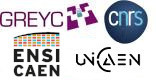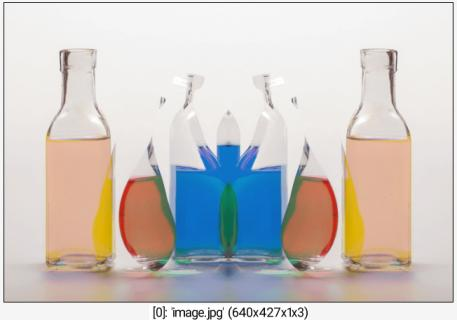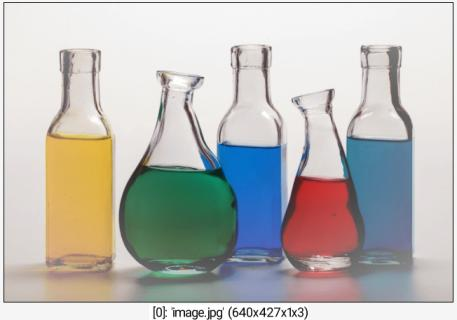G'MIC - GREYC's Magic for Image Computing: A Full-Featured Open-Source Framework for Image Processing# A Full-Featured Open-Source Framework for Image Processing

## Latest stable version: 3.1.4

Reference

 Table of Contents  ▸  List of Commands  ▸  Mathematical Operators  ▸  max ◀  log2    |    maxabs  ▶

# max

Built-in command

## Arguments:

• value[%]    or
• [image]    or
• 'formula'    or
• (no arg)

## Description:

Compute the maximum between selected images and specified value, image or mathematical expression, or compute the pointwise maxima between selected images.

## Examples of use:

### • Example #1

\$ gmic image.jpg +mirror x maxCommand: image.jpg +mirror x max

### • Example #2

\$ gmic image.jpg max 'R=((x/w-0.5)^2+(y/h-0.5)^2)^0.5;255*R'Command: image.jpg max 'R=((x/w-0.5)^2+(y/h-0.5)^2)^0.5;255*R'
G'MIC - GREYC's Magic for Image Computing: A Full-Featured Open-Source Framework for Image Processing